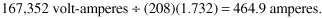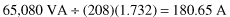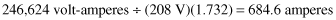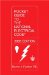# Example No. D5(b) Optional Calculation for Multifamily Dwelling Served at 208Y/120 Volts, Three Phase

### Example No D5(b) Optional Calculation for Multifamily Dwelling Served at 208Y 120 Volts, Three Phase

All conditions and calculations the same as for Optional Calculations for Multifamily Dwelling of Example No. D4(b) served at 120/40 volt, single phase except service to each dwelling unit shall be two-phase legs and neutral.

Minimum Number of Branch Circuits Required for Each Dwelling Unit (See 210.11)

Range circuit (see Table 220.55, Column B): 8000 volt-amperes x 80% ÷ 208 volt = 30.7 amperes or a circuit of two 8 AWG conductors and one 10 AWG conductor per 220.19(A)(3).

Space heating: 6000 volt-amperes ÷ 208 volts = 28.8 amperes. Two 20-ampere, 2-pole circuits required, 12 AWG conductors.

Minimum Size Feeder Required for Each Dwelling Unit

 120/208-volt, 3-wire circuit Net calculated load: 18,782 volt-amperes ÷ 208 volts = 90.3 amperes Net calculated lighting load (line to neutral): 3882 volt-amperes ÷ 2 legs ÷ 120 volts/leg = 16.2 amperes Line to line = 14,900 volt-amperes ÷ 208 volts 71.6 amperes Total load = 16.2 A + 71.6 A = 87.8 amperes

Minimum Size Feeder Required for Service Equipment to Meter Bank (20 Dwelling Units)Minimum Size Main Feeder Required (Less House Load) (40 Dwelling Units)Further Demand Factor [See 220.61(B)] 200 amperes at 100% 200.0 A 298A - 200A = 98.8 amperes at 70% 69.2 A Net computed load (neutral) 269.2 APocket Guide to the National Electrical Code(R), 2005 Edition (8th Edition)
ISBN: 0131480014
EAN: 2147483647
Year: 2004
Pages: 120